# Java Program to Print Pyramid Pattern of Stars

This article covers some programs that prints pyramid pattern of stars (*) in Java programming. Here are the list of programs covered in this article:

• Pyramid pattern of stars
• Inverted pyramid pattern of stars
• Half-pyramid pattern of stars
• Inverted half-pyramid pattern of stars
• Complete or full pyramid pattern of stars

## Pyramid Pattern of Stars (*) in Java

The question is, write a Java program to print pyramid pattern of stars (*). The answer to this question is the program given below:

```public class CodesCracker
{
public static void main(String[] args)
{
int row=9, i, space, j;
for(i=0; i<row; i++)
{
for(space=i; space<row; space++)
System.out.print(" ");
for(j=0; j<(i+1); j++)
System.out.print("* ");
System.out.print("\n");
}
}
}```

The output produced by above Java program is: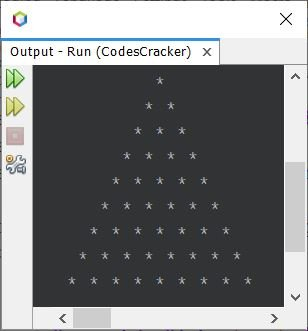### Java Code to Print Pyramid of Stars of Given Size

Let's modify the above program, to allow user to define the size of pyramid at run-time of the program:

```import java.util.Scanner;

public class CodesCracker
{
public static void main(String[] args)
{
int row, i, space, j;
Scanner s = new Scanner(System.in);

System.out.print("Enter the Number of Rows (Line): ");
row = s.nextInt();

for(i=0; i<row; i++)
{
for(space=i; space<row; space++)
System.out.print(" ");
for(j=0; j<(i+1); j++)
System.out.print("* ");
System.out.print("\n");
}
}
}```

The snapshot given below shows the sample run of above Java program with user input 12 as number of rows or lines to print pyramid pattern of given size: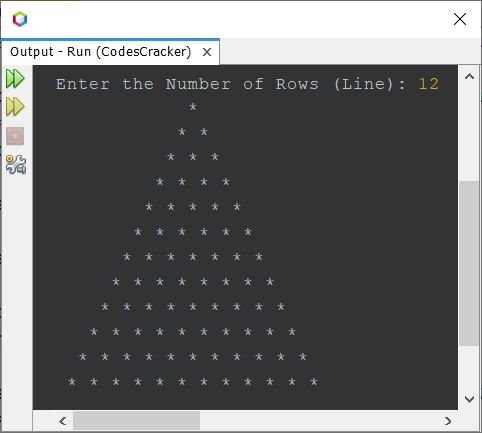## Inverted Pyramid Pattern of Stars in Java

To print inverted pyramid pattern of stars, use the program given below. To increase or decrease the row size of the pattern, just increase/decrease the value of row variable.

```public class CodesCracker
{
public static void main(String[] args)
{
int i, j, row=9, space;

for(i=0; i<row; i++)
{
for(space=0; space<i; space++)
System.out.print(" ");
for(j=i; j<row; j++)
System.out.print("* ");
System.out.print("\n");
}
}
}```

Here is its sample output: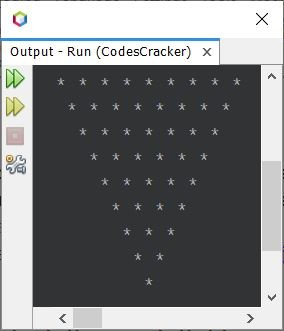## Half Pyramid Pattern of Stars in Java

This program prints half-pyramid pattern of stars in Java.

```public class CodesCracker
{
public static void main(String[] args)
{
int row=9, i, j;
for(i=0; i<row; i++)
{
for(j=0; j<=i; j++)
System.out.print("* ");
System.out.print("\n");
}
}
}```

#### Output of Previous Program - Half Pyramid Program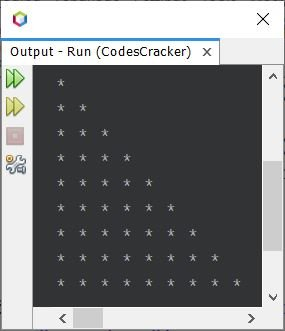## Inverted Half Pyramid Pattern of Stars in Java

Here is another program, to print inverted half pyramid of stars.

```public class CodesCracker
{
public static void main(String[] args)
{
int row=9, i, j;
for(i=0; i<row; i++)
{
for(j=i; j<row; j++)
System.out.print("* ");
System.out.print("\n");
}
}
}```

#### Output of Previous Program - Inverted Half Pyramid Program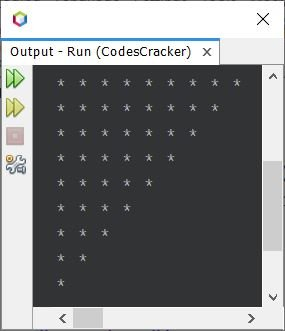## Complete Pyramid Pattern of Stars (*) in Java

This is the program I think we need, when we want to print full pyramid pattern using stars. Therefore, I've created the pyramid, that provides almost the actual looks of pyramid.

```import java.util.Scanner;

public class CodesCracker
{
public static void main(String[] args)
{
int i, row, space, j;
Scanner s = new Scanner(System.in);

System.out.print("Enter the Number of Rows (Line): ");
row = s.nextInt();

for(i=0; i<row; i++)
{
for(space=i; space<row; space++)
System.out.print(" ");
for(j=0; j<(i+1); j++)
System.out.print("* ");
System.out.print("\n");
}
for(i=row; i>0; i=(i-2))
{
for(space=row; space>=(i-1); space--)
System.out.print(" ");
for(j=(i-1); j>0; j--)
System.out.print("* ");
System.out.print("\n");
}
}
}```

Here is its sample run with user input 14 as number of rows to form the full pyramid of stars: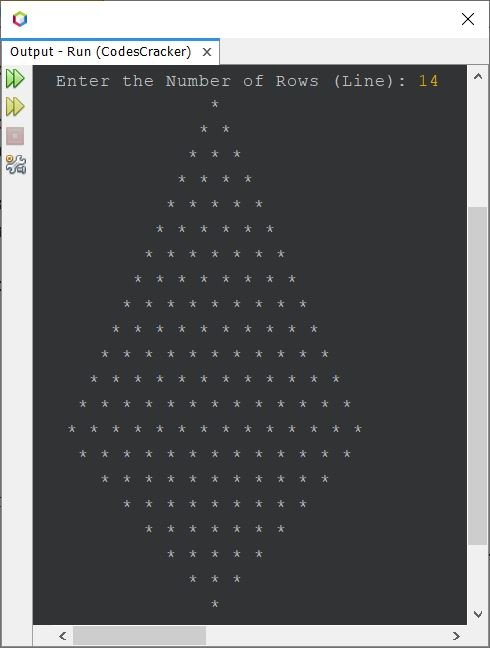#### Same Program in Other Languages

Java Online Test

« Previous Program Next Program »